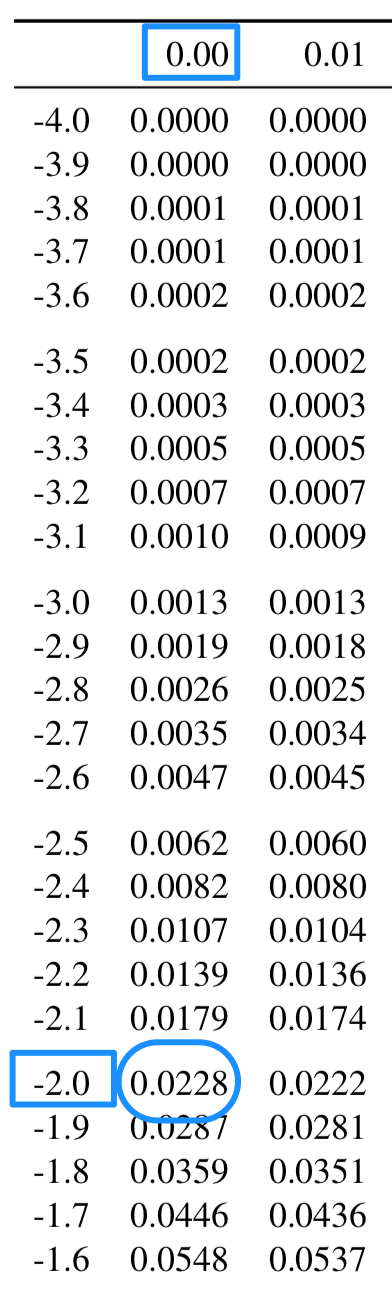## 17.6 Exact areas from normal distributions

Areas under normal distributions can be found using:

### 17.6.1 Using the online tables

The online tables work differently to the hard-copy tables. Consider the same example again: the percentage of observations smaller than $$z = -2$$.

Like the hard-copy tables, the online tables (Appendix B.2) work with two decimal places, so consider the $$z$$-score as $$z=-2.00$$. In the tables, enter the value -2 in the search region just under the column labelled z.score (see the animation below). After pressing Enter, the answer is shown in the column headed Area.to.left: the probability of finding a $$z$$-score less than $$-2$$ is 0.0228, or about 2.28%.

Using either the hard-copy or online tables gives an answer of about 2.28%. Using the 68–95–99.7 rule, the answer we obtained was $$2.5$$%. Recall that the 68–95–99.7 rule is an approximation only.

### 17.6.2 Using the hard-copy tables

To demonstration the use of the normal distribution tables, consider the percentage of observations smaller than $$z = -2$$ (that is, two standard deviations below the mean) in a normal distribution.

The hard-copy tables work with $$z$$-scores to two decimal places, so consider the $$z$$-score as $$z=-2.00$$. On the tables, find $$-2.0$$ in the left margin of the table, and find the second decimal place (in this case, 0) in the top margin of the table (Fig. 17.6): where these intersect is the area (or probability) less than the $$z$$-score. So the probability of finding a $$z$$-score less than $$z = -2$$ is 0.0228, or about 2.28%. (The online tables work differently.)FIGURE 17.6: Using the hard-copy tables to compute the probability that $$z$$ is less than $$-2$$

The tables give the area to the left of the $$z$$-score that is looked up.